# Circle Passing Through 3 Points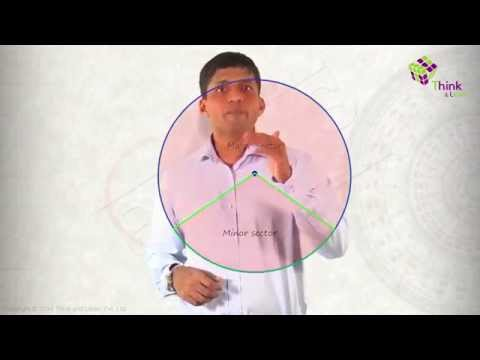To draw a straight line the minimum number of points required is two. Given any two points, a straight line can be drawn. How many minimum points are sufficient to draw a unique circle? Well, let’s try to find out.

## Circle Passing Through a Point

Let us consider a point and try to draw circle passing through that point.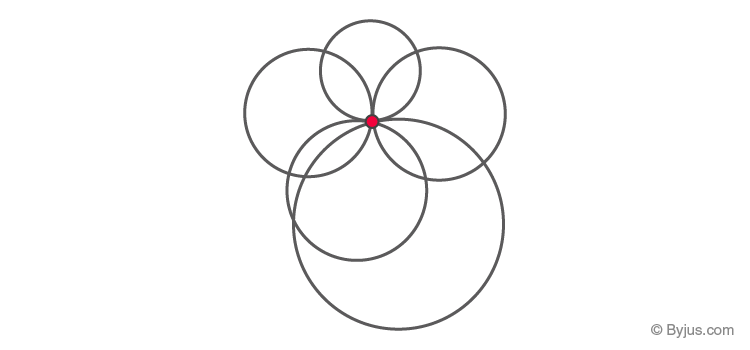It can be seen that through a single point P infinite circles passing through it can be drawn.

## Circle Passing Through Two Points

Now, let us take two points P and Q and see what happens?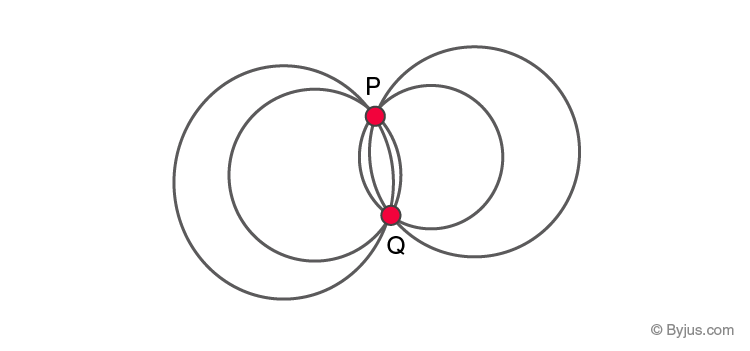Again we see that an infinite number of circles passing through points P and Q can be drawn.

## Circle Passing Through Three Points (Collinear or Non-Collinear)

Let us now take 3 points. For a circle passing through 3 points, two cases can arise.

• Three points can be collinear
• Three points can be non-collinear

Let us study both cases individually.

### Case 1: A circle passing through 3 points: Points are collinear

Consider three points P, Q and R which are collinear.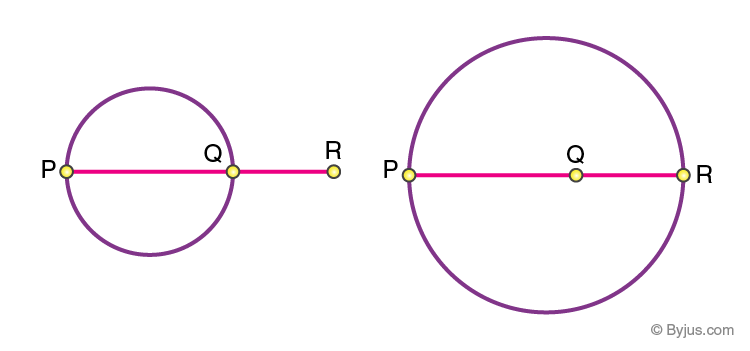It can be seen that if three points are collinear any one of the points either lie outside the circle or inside it. Therefore, a circle passing through 3 points, where the points are collinear is not possible.

### Case 2: A circle passing through 3 points: Points are non-collinear

To draw a circle through three non-collinear points join the points as shown: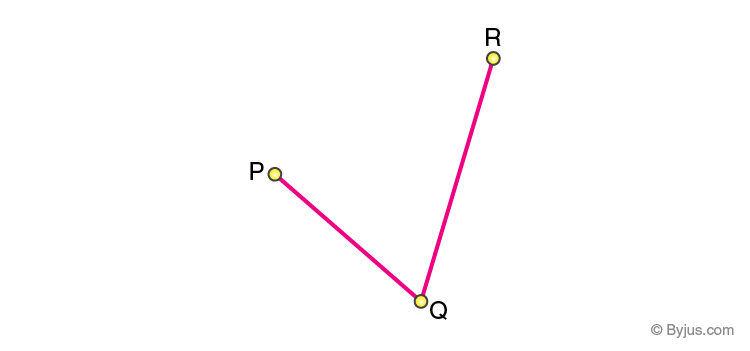Draw perpendicular bisectors of PQ and RQ. Let the bisectors AB and CD meet at O.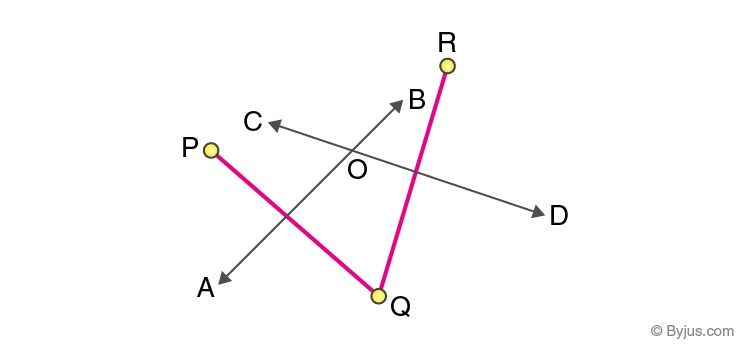With O as the centre and radius OP or OQ or OR draw a circle. We get a circle passing through 3 point P, Q, and R.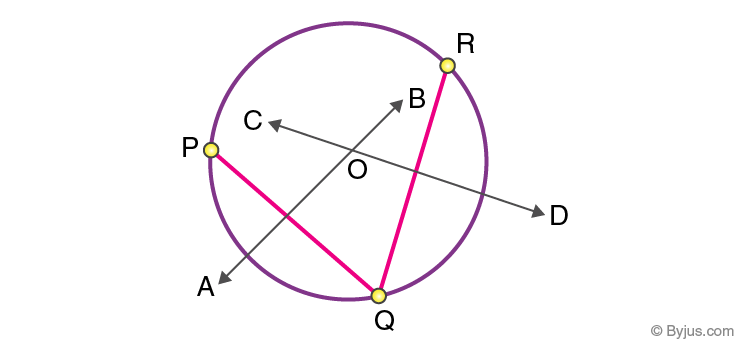It is observed that only a unique circle will pass through all three points. It can be stated as a theorem and the proof is explained as follows.

Given: Three non-collinear points A, B and C

To prove: One and only one circle can be drawn through A, B, and C

Construction: Join AB and BC. Draw perpendicular bisectors of AB and BC. Let these perpendicular bisectors meet at a point O.

### Proof: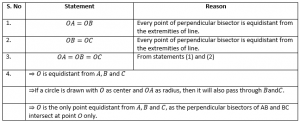From above it follows that a unique circle passing through 3 points can be drawn given that the points are non-collinear. To know more about the area of a circle, equation of a circle, and its properties download BYJU’S-The Learning App.